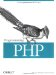14.9 References

 References at the PHP source level map fairly straightforwardly onto the internals. Consider this PHP code: Here \$b is a reference to the same zval container as \$a. Internally in PHP, the is_ref indicator is set to 1 for both the zval containers, and the reference count is set to 2. If the user then does an unset(\$b), the is_ref indicator on the \$a container is set to 0. The reference count actually remains at 2, since the \$a symbol table entry is still referring to this zval container and the zval container itself also counts as a reference when the container is not a reference itself (indicated by the is_ref flag being on). This may be a little bit confusing, but keep reading. When you allocate a new zval container using MAKE_STD_ZVAL( ), or if you call INIT_PZVAL( ) directly on a new container, the reference count is initialized to 1 and is_ref is set to 0. If a symbol table entry is then created for this container, the reference count becomes 2. If a second symbol table alias is created for this same container, the is_ref indicator is turned on. If a third symbol table alias is created for the container, the reference count on the container jumps to 3. A zval container can have a reference count greater than 1 without is_ref being turned on. This is for performance reasons. Say you want to write a function that creates an n-element array and initializes each element to a given value that you provide, much like PHP's array_fill( ) function. The code would look something like this: PHP_FUNCTION(foo) { long n; zval *val; int argc = ZEND_NUM_ARGS( ); if (zend_parse_parameters(argc TSRMLS_CC, "lz", &n, &val) == FAILURE) return; SEPARATE_ZVAL(&val); array_init(return_value); while(n--) { zval_add_ref(&val); add_next_index_zval(return_value, val); } } The function takes an integer and a raw zval (meaning that the second parameter to the function can be of any type). It then makes a copy of the passed zval container using SEPARATE_ZVAL( ), initializes the return_value to be an array, and fills in the array. The big trick here is the zval_add_ref( ) call. This function increments the reference count on the zval container. Therefore, instead of making n copies of the container, one for each element, we have only one copy, with a reference count of n+1. Remember, is_ref is still 0 here. Here's how this function could be used in a PHP script: This would result in a two-dimensional array that looks like this: \$arr = 1 \$arr = 2 \$arr = 3 \$arr = 1 \$arr = 2 \$arr = 3 \$arr = 1 \$arr = 2 \$arr = 3 Internally, a copy-on-write of the appropriate container is done if any of these array elements are changed. The engine knows to do a copy-on-write when it sees something being assigned to a zval container whose reference count is greater than 1 and whose is_ref is 0. We could have written our function to do a MAKE_STD_ZVAL( ) for each element in our array, but it would have been about twice as slow as simply incrementing the reference count and letting a copy-on-write make a separate copy later if necessary.Programming PHP
ISBN: 1565926102
EAN: 2147483647
Year: 2007
Pages: 168

Similar book on Amazon